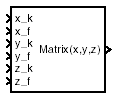# Interpolate Matrix(x,y,z)

Return interpolated matrix for given inputs

•Libraries:
Aerospace Blockset / GNC / Control

## Description

The Interpolate Matrix(x,y,z) block interpolates a three-dimensional array of matrices.

This three-dimensional case assumes the matrix is defined as a function of three independent variables:

x = [ x 1 x 2 x 3 ... x i x i+1 ... x n ]

y = [ y 1 y 2 y 3 ... y j y j+1 ... y m ]

z = [ z 1 z 2 z 3 ... z k z k+1 ... z p ]

For given values of x, y, and z, eight matrices are interpolated. Then for

x i < x < x i+1

y j < y < y j+1

z k < z < z k+1

the output matrix is given by

where the three interpolation fractions are denoted by

`$\begin{array}{l}{\lambda }_{x}=\left(x-{x}_{i}\right)/\left({x}_{i+1}-{x}_{i}\right)\\ {i}_{y}=\left(y-{y}_{j}\right)/\left({y}_{j+1}-{y}_{j}\right)\\ {\lambda }_{z}=\left(z-{z}_{k}\right)/\left({z}_{k+1}-{z}_{k}\right)\end{array}$`

In the three-dimensional case, the interpolation is carried out first on x, then y, and finally z.

The matrix to be interpolated should be five-dimensional, the first two dimensions corresponding to the matrix at each value of x, y, and z. For example, if you have eight matrices A, B, C, D, E, F, G, and H defined at the following values of x, y, and z, then the corresponding input matrix is given by

 `(x = 0.0,y = 1.0,z = 0.1)` `matrix(:,:,1,1,1) = A;` `(x = 0.0,y = 1.0,z = 0.5)` `matrix(:,:,1,1,2) = B;` `(x = 0.0,y = 3.0,z = 0.1) ` `matrix(:,:,1,2,1) = C;` `(x = 0.0,y = 3.0,z = 0.5) ` `matrix(:,:,1,2,2) = D;` `(x = 1.0,y = 1.0,z = 0.1)` `matrix(:,:,2,1,1) = E;` `(x = 1.0,y = 1.0,z = 0.5) ` `matrix(:,:,2,1,2) = F;` `(x = 1.0,y = 3.0,z = 0.1)` `matrix(:,:,2,2,1) = G;` `(x = 1.0,y = 3.0,z = 0.5)` `matrix(:,:,2,2,2) = H;`

## Limitations

This block must be driven from the Prelookup block.

## Ports

### Input

expand all

First interpolation index i, specified as a scalar.

Data Types: `double`

First interpolation fraction λ x, specified as a scalar .

Data Types: `double`

Second interpolation index j, specified as a scalar.

Data Types: `double`

Second interpolation fraction λ y, specified as a scalar.

Data Types: `double`

Third interpolation index k, specified as a scalar.

Data Types: `double`

Third interpolation fraction λ z, specified as a scalar.

Data Types: `double`

### Output

expand all

Interpolated matrix, specified as a matrix.

Data Types: `double`

## Parameters

expand all

Matrix to be interpolated, with five indices and the third, fourth, and fifth indices labeling the interpolating values of x, y, and z.

#### Programmatic Use

 Block Parameter: `matrix` Type: character vector Values: matrix Default: `'matrix'`

## Version History

Introduced before R2006a